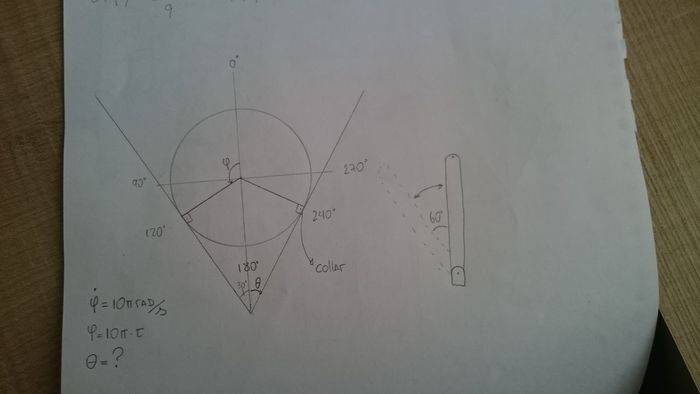# Conversion between two Harmonic Angular Motion

• DFornazari

## Homework Statement

I need to find a way to do a conversion between the angular motion of a motor to the angular motion of an oscilating bar that is connected to it through a sliding and rotating collar. This way, every time the motor completes a revolution, the bar swings back and forth with a amplitude of 30 degrees in 0,2s (2x30º = 60º). The problem is that I can't find an equation to compare both motions.

The motor angular velocity is 10π rad*s-1

## Homework Equations

SHM equations (maybe?)

## The Attempt at a Solution

I've tried using Angular Simple Harmonic Motion, but I think that as I'm working with big angles, the error will be way too big. I also tried comparing the angles of both motions in a table (Motor Angle [0º,360º] x Bar Angle[-30º,30º]), and found an equation: θ(φ) = sin(φ)*π*sqrt(3)/9. The problem is that this equation works when you use φ = 0, 120, 180 and 240, but it does not appear to work when angles between those are used. I think that happens because the relation is not linear due to acceleration.This is just a geometry problem. Your diagram shows the bar at one extreme of movement. Draw another with it in general position. What equation relates φ and θ?